# Problem 53940. Easy Sequences 66: Fractional Part of Sum of Consecutive Powers over a Prime

Given three postive integers, x, e and n, create the function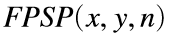, defined as follows:Where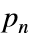is the nth prime and the symbol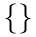is the fractional part function of a positive real number, defined as follows:
If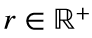then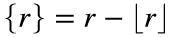, where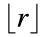, is the 'floor' of r.
For example for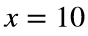,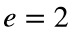and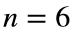, we have:Therefore, since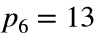:Please round-off the output to four decimal places, so in this case: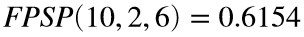.
HINT: This is related to the Faulhaber's formula.

### Solution Stats

60.0% Correct | 40.0% Incorrect
Last Solution submitted on Sep 06, 2023

### Community Treasure Hunt

Find the treasures in MATLAB Central and discover how the community can help you!

Start Hunting!